GFG App
Open AppBrowser
Continue

# Reverse Delete Algorithm for Minimum Spanning Tree

Reverse Delete algorithm is closely related to Kruskal’s algorithm. In Kruskal’s algorithm what we do is : Sort edges by increasing order of their weights. After sorting, we one by one pick edges in increasing order. We include current picked edge if by including this in spanning tree not form any cycle until there are V-1 edges in spanning tree, where V = number of vertices.

In Reverse Delete algorithm, we sort all edges in decreasing order of their weights. After sorting, we one by one pick edges in decreasing order. We include current picked edge if excluding current edge causes disconnection in current graph. The main idea is delete edge if its deletion does not lead to disconnection of graph.

The Algorithm :

1. Sort all edges of graph in non-increasing order of edge weights.
2. Initialize MST as original graph and remove extra edges using step 3.
3. Pick highest weight edge from remaining edges and check if deleting the edge disconnects the graph   or not.
If disconnects, then we don’t delete the edge.
Else we delete the edge and continue.

Illustration:

Let us understand with the following example: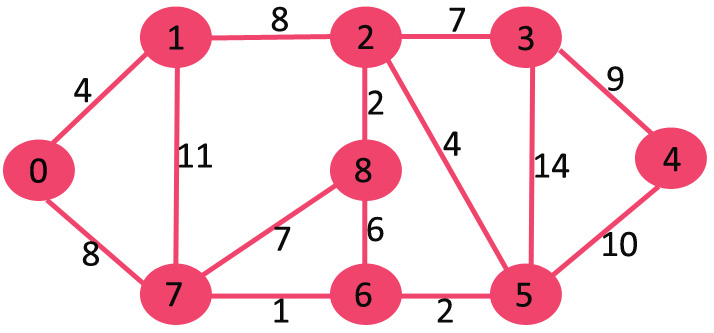If we delete highest weight edge of weight 14, graph doesn’t become disconnected, so we remove it.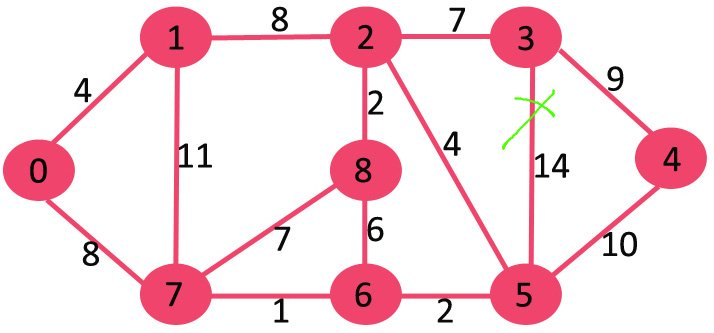Next we delete 11 as deleting it doesn’t disconnect the graph.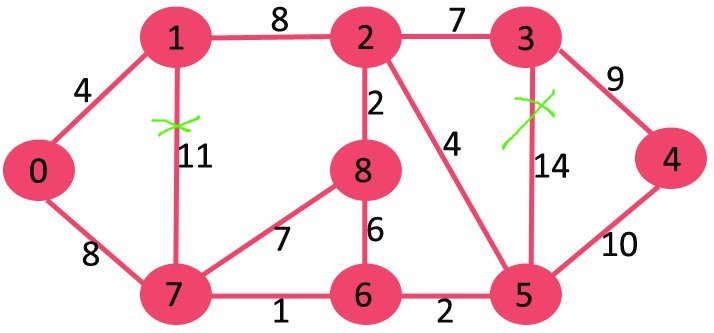Next we delete 10 as deleting it doesn’t disconnect the graph.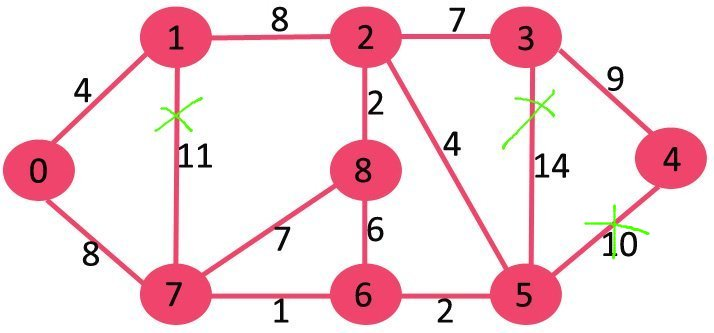Next is 9. We cannot delete 9 as deleting it causes disconnection.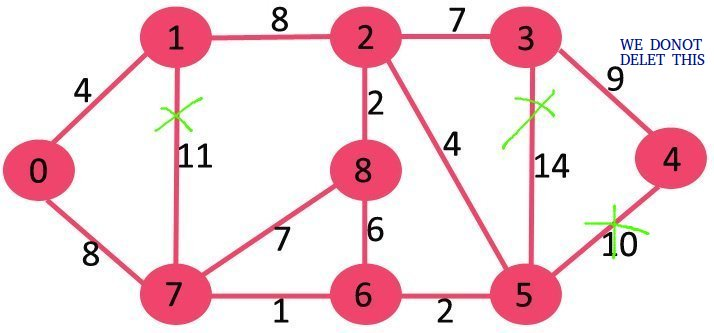We continue this way and following edges remain in final MST.

```Edges in MST
(3, 4)
(0, 7)
(2, 3)
(2, 5)
(0, 1)
(5, 6)
(2, 8)
(6, 7) ```

Note : In case of same weight edges, we can pick any edge of the same weight edges.

Implementation:

## C++

 `// C++ program to find Minimum Spanning Tree` `// of a graph using Reverse Delete Algorithm` `#include` `using` `namespace` `std;`   `// Creating shortcut for an integer pair` `typedef`  `pair<``int``, ``int``> iPair;`   `// Graph class represents a directed graph` `// using adjacency list representation` `class` `Graph` `{` `    ``int` `V;    ``// No. of vertices` `    ``list<``int``> *adj;` `    ``vector< pair<``int``, iPair> > edges;` `    ``void` `DFS(``int` `v, ``bool` `visited[]);`   `public``:` `    ``Graph(``int` `V);   ``// Constructor`   `    ``// function to add an edge to graph` `    ``void` `addEdge(``int` `u, ``int` `v, ``int` `w);`   `    ``// Returns true if graph is connected` `    ``bool` `isConnected();`   `    ``void` `reverseDeleteMST();` `};`   `Graph::Graph(``int` `V)` `{` `    ``this``->V = V;` `    ``adj = ``new` `list<``int``>[V];` `}`   `void` `Graph::addEdge(``int` `u, ``int` `v, ``int` `w)` `{` `    ``adj[u].push_back(v); ``// Add w to v’s list.` `    ``adj[v].push_back(u); ``// Add w to v’s list.` `    ``edges.push_back({w, {u, v}});` `}`   `void` `Graph::DFS(``int` `v, ``bool` `visited[])` `{` `    ``// Mark the current node as visited and print it` `    ``visited[v] = ``true``;`   `    ``// Recur for all the vertices adjacent to` `    ``// this vertex` `    ``list<``int``>::iterator i;` `    ``for` `(i = adj[v].begin(); i != adj[v].end(); ++i)` `        ``if` `(!visited[*i])` `            ``DFS(*i, visited);` `}`   `// Returns true if given graph is connected, else false` `bool` `Graph::isConnected()` `{` `    ``bool` `visited[V];` `    ``memset``(visited, ``false``, ``sizeof``(visited));`   `    ``// Find all reachable vertices from first vertex` `    ``DFS(0, visited);`   `    ``// If set of reachable vertices includes all,` `    ``// return true.` `    ``for` `(``int` `i=1; i=0; i--)` `    ``{` `        ``int` `u = edges[i].second.first;` `        ``int` `v = edges[i].second.second;`   `        ``// Remove edge from undirected graph` `        ``adj[u].``remove``(v);` `        ``adj[v].``remove``(u);`   `        ``// Adding the edge back if removing it` `        ``// causes disconnection. In this case this ` `        ``// edge becomes part of MST.` `        ``if` `(isConnected() == ``false``)` `        ``{` `            ``adj[u].push_back(v);` `            ``adj[v].push_back(u);`   `            ``// This edge is part of MST` `            ``cout << ``"("` `<< u << ``", "` `<< v << ``") \n"``;` `            ``mst_wt += edges[i].first;` `        ``}` `    ``}`   `    ``cout << ``"Total weight of MST is "` `<< mst_wt;` `}`   `// Driver code` `int` `main()` `{` `    ``// create the graph given in above figure` `    ``int` `V = 9;` `    ``Graph g(V);`   `    ``//  making above shown graph` `    ``g.addEdge(0, 1, 4);` `    ``g.addEdge(0, 7, 8);` `    ``g.addEdge(1, 2, 8);` `    ``g.addEdge(1, 7, 11);` `    ``g.addEdge(2, 3, 7);` `    ``g.addEdge(2, 8, 2);` `    ``g.addEdge(2, 5, 4);` `    ``g.addEdge(3, 4, 9);` `    ``g.addEdge(3, 5, 14);` `    ``g.addEdge(4, 5, 10);` `    ``g.addEdge(5, 6, 2);` `    ``g.addEdge(6, 7, 1);` `    ``g.addEdge(6, 8, 6);` `    ``g.addEdge(7, 8, 7);`   `    ``g.reverseDeleteMST();` `    ``return` `0;` `}`

## Java

 `// Java program to find Minimum Spanning Tree` `// of a graph using Reverse Delete Algorithm` `import` `java.util.*;`   `// class to represent an edge` `class` `Edge ``implements` `Comparable {` `  ``int` `u, v, w;` `  ``Edge(``int` `u, ``int` `v, ``int` `w)` `  ``{` `    ``this``.u = u;` `    ``this``.w = w;` `    ``this``.v = v;` `  ``}` `  ``public` `int` `compareTo(Edge other)` `  ``{` `    ``return` `(``this``.w - other.w);` `  ``}` `}` `// Class to represent a graph using adjacency list` `// representation` `public` `class` `GFG {`   `  ``private` `int` `V; ``// No. of vertices` `  ``private` `List[] adj;` `  ``private` `List edges;` `  ``@SuppressWarnings``({ ``"unchecked"``, ``"deprecated"` `})` `  ``public` `GFG(``int` `v) ``// Constructor` `  ``{` `    ``V = v;` `    ``adj = ``new` `ArrayList[v];` `    ``for` `(``int` `i = ``0``; i < v; i++)` `      ``adj[i] = ``new` `ArrayList();` `    ``edges = ``new` `ArrayList();` `  ``}`   `  ``// function to Add an edge` `  ``public` `void` `AddEdge(``int` `u, ``int` `v, ``int` `w)` `  ``{` `    ``adj[u].add(v); ``// Add w to v’s list.` `    ``adj[v].add(u); ``// Add w to v’s list.` `    ``edges.add(``new` `Edge(u, v, w));` `  ``}`   `  ``// function to perform dfs` `  ``private` `void` `DFS(``int` `v, ``boolean``[] visited)` `  ``{` `    ``// Mark the current node as visited and print it` `    ``visited[v] = ``true``;` `    ``// Recur for all the vertices adjacent to` `    ``// this vertex` `    ``for` `(``int` `i : adj[v]) {` `      ``if` `(!visited[i])` `        ``DFS(i, visited);` `    ``}` `  ``}` `  ``// Returns true if given graph is connected, else false` `  ``private` `boolean` `IsConnected()` `  ``{` `    ``boolean``[] visited = ``new` `boolean``[V];` `    ``// Find all reachable vertices from first vertex` `    ``DFS(``0``, visited);` `    ``// If set of reachable vertices includes all,` `    ``// return true.` `    ``for` `(``int` `i = ``1``; i < V; i++) {` `      ``if` `(visited[i] == ``false``)` `        ``return` `false``;` `    ``}` `    ``return` `true``;` `  ``}`   `  ``// This function assumes that edge (u, v)` `  ``// exists in graph or not,` `  ``public` `void` `ReverseDeleteMST()` `  ``{` `    ``// Sort edges in increasing order on basis of cost` `    ``Collections.sort(edges);` `    ``int` `mst_wt = ``0``; ``// Initialize weight of MST` `    ``System.out.println(``"Edges in MST"``);` `    ``// Iterate through all sorted edges in` `    ``// decreasing order of weights` `    ``for` `(``int` `i = edges.size() - ``1``; i >= ``0``; i--) {` `      ``int` `u = edges.get(i).u;` `      ``int` `v = edges.get(i).v;` `      ``// Remove edge from undirected graph` `      ``adj[u].remove(adj[u].indexOf(v));` `      ``adj[v].remove(adj[v].indexOf(u));`   `      ``// Adding the edge back if removing it` `      ``// causes disconnection. In this case this` `      ``// edge becomes part of MST.` `      ``if` `(IsConnected() == ``false``) {` `        ``adj[u].add(v);` `        ``adj[v].add(u);` `        ``// This edge is part of MST` `        ``System.out.println(``"("` `+ u + ``", "` `+ v` `                           ``+ ``")"``);` `        ``mst_wt += edges.get(i).w;` `      ``}` `    ``}` `    ``System.out.println(``"Total weight of MST is "` `                       ``+ mst_wt);` `  ``}` `  ``// Driver code` `  ``public` `static` `void` `main(String[] args)` `  ``{` `    ``// create the graph given in above figure` `    ``int` `V = ``9``;` `    ``GFG g = ``new` `GFG(V);` `    ``// making above shown graph` `    ``g.AddEdge(``0``, ``1``, ``4``);` `    ``g.AddEdge(``0``, ``7``, ``8``);` `    ``g.AddEdge(``1``, ``2``, ``8``);` `    ``g.AddEdge(``1``, ``7``, ``11``);` `    ``g.AddEdge(``2``, ``3``, ``7``);` `    ``g.AddEdge(``2``, ``8``, ``2``);` `    ``g.AddEdge(``2``, ``5``, ``4``);` `    ``g.AddEdge(``3``, ``4``, ``9``);` `    ``g.AddEdge(``3``, ``5``, ``14``);` `    ``g.AddEdge(``4``, ``5``, ``10``);` `    ``g.AddEdge(``5``, ``6``, ``2``);` `    ``g.AddEdge(``6``, ``7``, ``1``);` `    ``g.AddEdge(``6``, ``8``, ``6``);` `    ``g.AddEdge(``7``, ``8``, ``7``);`   `    ``g.ReverseDeleteMST();` `  ``}` `}`   `// This code is contributed by Prithi_Dey`

## Python3

 `# Python3 program to find Minimum Spanning Tree` `# of a graph using Reverse Delete Algorithm`   `# Graph class represents a directed graph` `# using adjacency list representation` `class` `Graph:` `    ``def` `__init__(``self``, v):`   `        ``# No. of vertices` `        ``self``.v ``=` `v` `        ``self``.adj ``=` `[``0``] ``*` `v` `        ``self``.edges ``=` `[]` `        ``for` `i ``in` `range``(v):` `            ``self``.adj[i] ``=` `[]`   `    ``# function to add an edge to graph` `    ``def` `addEdge(``self``, u: ``int``, v: ``int``, w: ``int``):` `        ``self``.adj[u].append(v) ``# Add w to v’s list.` `        ``self``.adj[v].append(u) ``# Add w to v’s list.` `        ``self``.edges.append((w, (u, v)))`   `    ``def` `dfs(``self``, v: ``int``, visited: ``list``):`   `        ``# Mark the current node as visited and print it` `        ``visited[v] ``=` `True`   `        ``# Recur for all the vertices adjacent to` `        ``# this vertex` `        ``for` `i ``in` `self``.adj[v]:` `            ``if` `not` `visited[i]:` `                ``self``.dfs(i, visited)`   `    ``# Returns true if graph is connected` `    ``# Returns true if given graph is connected, else false` `    ``def` `connected(``self``):` `        ``visited ``=` `[``False``] ``*` `self``.v`   `        ``# Find all reachable vertices from first vertex` `        ``self``.dfs(``0``, visited)`   `        ``# If set of reachable vertices includes all,` `        ``# return true.` `        ``for` `i ``in` `range``(``1``, ``self``.v):` `            ``if` `not` `visited[i]:` `                ``return` `False`   `        ``return` `True`   `    ``# This function assumes that edge (u, v)` `    ``# exists in graph or not,` `    ``def` `reverseDeleteMST(``self``):`   `        ``# Sort edges in increasing order on basis of cost` `        ``self``.edges.sort(key ``=` `lambda` `a: a[``0``])`   `        ``mst_wt ``=` `0` `# Initialize weight of MST`   `        ``print``(``"Edges in MST"``)`   `        ``# Iterate through all sorted edges in` `        ``# decreasing order of weights` `        ``for` `i ``in` `range``(``len``(``self``.edges) ``-` `1``, ``-``1``, ``-``1``):` `            ``u ``=` `self``.edges[i][``1``][``0``]` `            ``v ``=` `self``.edges[i][``1``][``1``]`   `            ``# Remove edge from undirected graph` `            ``self``.adj[u].remove(v)` `            ``self``.adj[v].remove(u)`   `            ``# Adding the edge back if removing it` `            ``# causes disconnection. In this case this` `            ``# edge becomes part of MST.` `            ``if` `self``.connected() ``=``=` `False``:` `                ``self``.adj[u].append(v)` `                ``self``.adj[v].append(u)`   `                ``# This edge is part of MST` `                ``print``(``"( %d, %d )"` `%` `(u, v))` `                ``mst_wt ``+``=` `self``.edges[i][``0``]` `        ``print``(``"Total weight of MST is"``, mst_wt)`   `# Driver Code` `if` `__name__ ``=``=` `"__main__"``:`   `    ``# create the graph given in above figure` `    ``V ``=` `9` `    ``g ``=` `Graph(V)`   `    ``# making above shown graph` `    ``g.addEdge(``0``, ``1``, ``4``)` `    ``g.addEdge(``0``, ``7``, ``8``)` `    ``g.addEdge(``1``, ``2``, ``8``)` `    ``g.addEdge(``1``, ``7``, ``11``)` `    ``g.addEdge(``2``, ``3``, ``7``)` `    ``g.addEdge(``2``, ``8``, ``2``)` `    ``g.addEdge(``2``, ``5``, ``4``)` `    ``g.addEdge(``3``, ``4``, ``9``)` `    ``g.addEdge(``3``, ``5``, ``14``)` `    ``g.addEdge(``4``, ``5``, ``10``)` `    ``g.addEdge(``5``, ``6``, ``2``)` `    ``g.addEdge(``6``, ``7``, ``1``)` `    ``g.addEdge(``6``, ``8``, ``6``)` `    ``g.addEdge(``7``, ``8``, ``7``)`   `    ``g.reverseDeleteMST()`   `# This code is contributed by` `# sanjeev2552`

## C#

 `// C# program to find Minimum Spanning Tree` `// of a graph using Reverse Delete Algorithm`   `using` `System;` `using` `System.Collections.Generic;`   `// class to represent an edge` `public` `class` `Edge : IComparable {` `    ``public` `int` `u, v, w;` `    ``public` `Edge(``int` `u, ``int` `v, ``int` `w)` `    ``{` `        ``this``.u = u;` `        ``this``.v = v;` `        ``this``.w = w;` `    ``}` `    ``public` `int` `CompareTo(Edge other)` `    ``{` `        ``return` `this``.w.CompareTo(other.w);` `    ``}` `}`   `// Graph class represents a directed graph` `// using adjacency list representation` `public` `class` `Graph {` `    ``private` `int` `V; ``// No. of vertices` `    ``private` `List<``int``>[] adj;` `    ``private` `List edges;`   `    ``public` `Graph(``int` `v) ``// Constructor` `    ``{` `        ``V = v;` `        ``adj = ``new` `List<``int``>[ v ];` `        ``for` `(``int` `i = 0; i < v; i++)` `            ``adj[i] = ``new` `List<``int``>();` `        ``edges = ``new` `List();` `    ``}`   `    ``// function to Add an edge` `    ``public` `void` `AddEdge(``int` `u, ``int` `v, ``int` `w)` `    ``{` `        ``adj[u].Add(v); ``// Add w to v’s list.` `        ``adj[v].Add(u); ``// Add w to v’s list.` `        ``edges.Add(``new` `Edge(u, v, w));` `    ``}`   `    ``// function to perform dfs` `    ``private` `void` `DFS(``int` `v, ``bool``[] visited)` `    ``{` `        ``// Mark the current node as visited and print it` `        ``visited[v] = ``true``;`   `        ``// Recur for all the vertices adjacent to` `        ``// this vertex` `        ``foreach``(``int` `i ``in` `adj[v])` `        ``{` `            ``if` `(!visited[i])` `                ``DFS(i, visited);` `        ``}` `    ``}`   `    ``// Returns true if given graph is connected, else false` `    ``private` `bool` `IsConnected()` `    ``{` `        ``bool``[] visited = ``new` `bool``[V];`   `        ``// Find all reachable vertices from first vertex` `        ``DFS(0, visited);`   `        ``// If set of reachable vertices includes all,` `        ``// return true.` `        ``for` `(``int` `i = 1; i < V; i++) {` `            ``if` `(visited[i] == ``false``)` `                ``return` `false``;` `        ``}` `        ``return` `true``;` `    ``}`   `    ``// This function assumes that edge (u, v)` `    ``// exists in graph or not,` `    ``public` `void` `ReverseDeleteMST()` `    ``{` `        ``// Sort edges in increasing order on basis of cost` `        ``edges.Sort();`   `        ``int` `mst_wt = 0; ``// Initialize weight of MST`   `        ``Console.WriteLine(``"Edges in MST"``);`   `        ``// Iterate through all sorted edges in` `        ``// decreasing order of weights` `        ``for` `(``int` `i = edges.Count - 1; i >= 0; i--) {` `            ``int` `u = edges[i].u;` `            ``int` `v = edges[i].v;`   `            ``// Remove edge from undirected graph` `            ``adj[u].Remove(v);` `            ``adj[v].Remove(u);`   `            ``// Adding the edge back if removing it` `            ``// causes disconnection. In this case this` `            ``// edge becomes part of MST.` `            ``if` `(IsConnected() == ``false``) {` `                ``adj[u].Add(v);` `                ``adj[v].Add(u);`   `                ``// This edge is part of MST` `                ``Console.WriteLine(``"({0}, {1})"``, u, v);` `                ``mst_wt += edges[i].w;` `            ``}` `        ``}`   `        ``Console.WriteLine(``"Total weight of MST is {0}"``,` `                          ``mst_wt);` `    ``}` `}`   `class` `GFG {` `    ``// Driver code` `    ``static` `void` `Main(``string``[] args)` `    ``{` `        ``// create the graph given in above figure` `        ``int` `V = 9;` `        ``Graph g = ``new` `Graph(V);`   `        ``// making above shown graph` `        ``g.AddEdge(0, 1, 4);` `        ``g.AddEdge(0, 7, 8);` `        ``g.AddEdge(1, 2, 8);` `        ``g.AddEdge(1, 7, 11);` `        ``g.AddEdge(2, 3, 7);` `        ``g.AddEdge(2, 8, 2);` `        ``g.AddEdge(2, 5, 4);` `        ``g.AddEdge(3, 4, 9);` `        ``g.AddEdge(3, 5, 14);` `        ``g.AddEdge(4, 5, 10);` `        ``g.AddEdge(5, 6, 2);` `        ``g.AddEdge(6, 7, 1);` `        ``g.AddEdge(6, 8, 6);` `        ``g.AddEdge(7, 8, 7);`   `        ``g.ReverseDeleteMST();` `    ``}` `}`   `// This code is contributed by cavi4762`

## Javascript

 `// Javascript program to find Minimum Spanning Tree` `// of a graph using Reverse Delete Algorithm`   `// Graph class represents a directed graph` `// using adjacency list representation` `class Graph {`   `    ``// Constructor` `    ``constructor(V) {` `        ``this``.V = V;` `        ``this``.adj = [];` `        ``this``.edges = [];` `        ``for` `(let i = 0; i < V; i++) {` `            ``this``.adj[i] = [];` `        ``}` `    ``}` `    `  `    ``// function to add an edge to graph` `    ``addEdge(u, v, w) {` `        ``this``.adj[u].push(v);``// Add w to v’s list.` `        ``this``.adj[v].push(u);``// Add w to v’s list.` `        ``this``.edges.push([w, [u, v]]);` `    ``}`   `    ``DFS(v, visited) {` `        ``// Mark the current node as visited and print it` `        ``visited[v] = ``true``;` `        ``for` `(const i of ``this``.adj[v]) {` `            ``if` `(!visited[i]) {` `                ``this``.DFS(i, visited);` `            ``}` `        ``}` `    ``}`   `    ``// Returns true if given graph is connected, else false` `    ``isConnected() {` `        ``const visited = [];` `        ``for` `(let i = 0; i < ``this``.V; i++) {` `            ``visited[i] = ``false``;` `        ``}` `        `  `        ``// Find all reachable vertices from first vertex` `        ``this``.DFS(0, visited);` `        `  `        ``// If set of reachable vertices includes all,` `        ``// return true.` `        ``for` `(let i = 1; i < ``this``.V; i++) {` `            ``if` `(!visited[i]) {` `                ``return` `false``;` `            ``}` `        ``}` `        ``return` `true``;` `    ``}`   `    ``// This function assumes that edge (u, v)` `    ``// exists in graph or not,` `    ``reverseDeleteMST() {` `    `  `        ``// Sort edges in increasing order on basis of cost` `        ``this``.edges.sort((a, b) => a - b);` `        `  `        ``let mstWt = 0;``// Initialize weight of MST` `        `  `        ``console.log(``"Edges in MST"``);` `        `  `        ``// Iterate through all sorted edges in` `        ``// decreasing order of weights` `        ``for` `(let i = ``this``.edges.length - 1; i >= 0; i--) {` `            ``const [u, v] = ``this``.edges[i];` `            `  `            ``// Remove edge from undirected graph` `            ``this``.adj[u] = ``this``.adj[u].filter(x => x !== v);` `            ``this``.adj[v] = ``this``.adj[v].filter(x => x !== u);` `            `  `            ``// Adding the edge back if removing it` `            ``// causes disconnection. In this case this ` `            ``// edge becomes part of MST.` `            ``if` `(!``this``.isConnected()) {` `                ``this``.adj[u].push(v);` `                ``this``.adj[v].push(u);` `                `  `                ``// This edge is part of MST` `                ``console.log(`(\${u}, \${v})`);` `                ``mstWt += ``this``.edges[i];` `            ``}` `        ``}` `        ``console.log(`Total weight of MST is \${mstWt}`);` `    ``}` `}`   `// Driver code` `function` `main()` `{`   `    ``// create the graph given in above figure` `    ``var` `V = 9;` `    ``var` `g = ``new` `Graph(V);`   `    ``// making above shown graph` `    ``g.addEdge(0, 1, 4);` `    ``g.addEdge(0, 7, 8);` `    ``g.addEdge(1, 2, 8);` `    ``g.addEdge(1, 7, 11);` `    ``g.addEdge(2, 3, 7);` `    ``g.addEdge(2, 8, 2);` `    ``g.addEdge(2, 5, 4);` `    ``g.addEdge(3, 4, 9);` `    ``g.addEdge(3, 5, 14);` `    ``g.addEdge(4, 5, 10);` `    ``g.addEdge(5, 6, 2);` `    ``g.addEdge(6, 7, 1);` `    ``g.addEdge(6, 8, 6);` `    ``g.addEdge(7, 8, 7);`   `    ``g.reverseDeleteMST();` `}` `main();`

Output

```Edges in MST
(3, 4)
(0, 7)
(2, 3)
(2, 5)
(0, 1)
(5, 6)
(2, 8)
(6, 7)
Total weight of MST is 37```

Time complexity: O(E log E) where E is the number of edges.

Space complexity: O(V+E) where V is the number of vertices and E is the number of edges. We are using adjacency list to store the graph, so we need space proportional to O(V+E).

Notes :

1. The above implementation is a simple/naive implementation of Reverse Delete algorithm and can be optimized to O(E log V (log log V)3) [Source : Wiki]. But this optimized time complexity is still less than Prim and Kruskal Algorithms for MST.
2. The above implementation modifies the original graph. We can create a copy of the graph if original graph must be retained.

This article is contributed by Antra Purohit. If you like GeeksforGeeks and would like to contribute, you can also write an article using write.geeksforgeeks.org or mail your article to review-team@geeksforgeeks.org. See your article appearing on the GeeksforGeeks main page and help other Geeks.

My Personal Notes arrow_drop_up